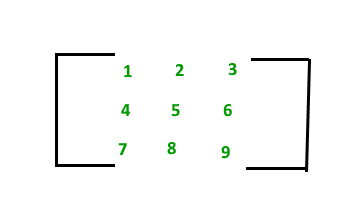# Matrix Data Structure

• Last Updated : 08 May, 2023

## What is Matrix Data Structure?

A matrix represents a collection of numbers arranged in an order of rows and columns. It is necessary to enclose the elements of a matrix in parentheses or brackets.
For example:

A matrix with 9 elements is shown below.This Matrix [M] has 3 rows and 3 columns. Each element of matrix [M] can be referred to by its row and column number. For example, a23 = 6.

Topics:

Introduction:

Basic Operations on Matrix:

Standard problems on Matrix: